# Chebyshev polynomials

Chebyshev polynomials of the first kind are a set of orthogonal polynomials defined as the solutions to the Chebyshev differential equation and denoted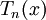$T_n(x)$. They are used as an approximation to a least squares fit, and are a special case of the ultra-spherical polynomial (Gegenbauer polynomial) with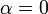$\alpha=0$. Chebyshev polynomial of the first kind,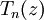$T_n (z)$ can be defined by the contour integral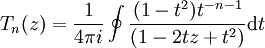$T_n (z) = \frac{1}{4 \pi i} \oint \frac{(1-t^2)t^{-n-1}}{(1-2tz+t^2)} {\rm d}t$

The first seven Chebyshev polynomials of the first kind are: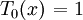$\left. T_0 (x) \right. =1$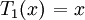$\left. T_1 (x) \right. =x$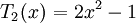$\left. T_2 (x) \right. =2x^2 -1$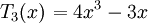$\left. T_3 (x) \right. =4x^3 - 3x$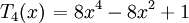$\left. T_4 (x) \right. =8x^4 - 8x^2 +1$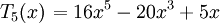$\left. T_5 (x) \right. =16x^5 - 20x^3 +5x$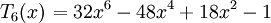$\left. T_6 (x)\right. =32x^6 - 48x^4 + 18x^2 -1$

## Orthogonality

The Chebyshev polynomials are orthogonal polynomials with respect to the weighting function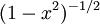$(1-x^2)^{-1/2}$ such that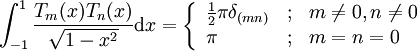$\int_{-1}^{1} \frac{T_m (x)T_n (x) }{ \sqrt{1-x^2}} \mathrm{d} x= \left\{ \begin{array}{lll} \frac{1}{2}\pi \delta_{(mn)} & ; & m \neq 0, n\neq 0 \\ \pi & ; & m=n=0 \end{array} \right.$

where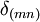$\delta_{(mn)}$ is the Kronecker delta.